# GANs from Scratch 1: A deep introduction. With code in PyTorch and TensorFlow

## “The coolest idea in deep learning in the last 20 years.” — Yann LeCun on GANs.

In this blog post we will explore Generative Adversarial Networks (GANs). If you haven’t heard of them before, this is your opportunity to learn all of what you’ve been missing out until now. If you’re not familiar with GANs, they’ve been hype during the last few years, specially the last semester. Though they’ve existed since 2014, GANs have already become widely known for their application versatility and their outstanding results in generating data.

They have been used in real-life applications for text/image/video generation, drug discovery and text-to-image synthesis. Just to give you an idea of their potential, here’s a short list of incredible projects created with GANs that you should definitely check out: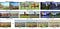Image-to-Image Translation using GANs. 

In this blog post we’ll start by describing Generative Algorithms and why GANs are becoming increasingly relevant. An overview and a detailed explanation on how and why GANs work will follow. Finally, we’ll be programming a Vanilla GAN, which is the first GAN model ever proposed! Feel free to read this blog in the order you prefer.

For demonstration purposes we’ll be using PyTorch, although a TensorFlow implementation can also be found in my GitHub Repo github.com/diegoalejogm/gans. You can check out some of the advanced GAN models (e.g. DCGAN) in the same GitHub repository if you’re interested, which by the way will also be explained in the series of posts that I’m starting, so make sure to stay tuned.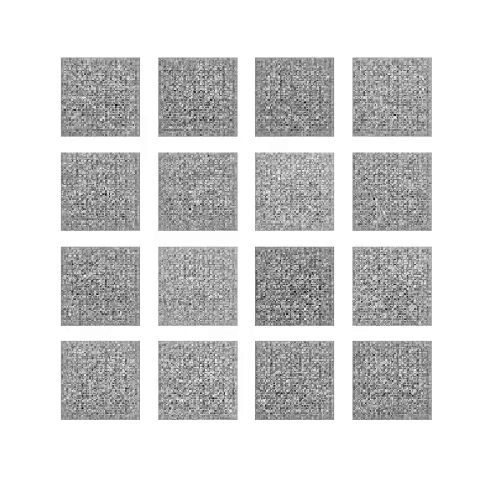Output of a GAN through time, learning to Create Hand-written digits. We’ll code this example!

# 1. Introduction

Generative Adversarial Networks (or GANs for short) are one of the most popular Machine Learning algorithms developed in recent times.

For those new to the field of Artificial Intelligence (AI), we can briefly describe Machine Learning (ML) as the sub-field of AI that uses data to “teach” a machine/program how to perform a new task. A simple example of this would be using images of a person’s face as input to the algorithm, so that a program learns to recognize that same person in any given picture (it’ll probably need negative samples too). For this purpose, we can describe Machine Learning as applied mathematical optimization, where an algorithm can represent data (e.g. a picture) in a multi-dimensional space (remember the Cartesian Plane? That’s a 2 dimensional field), and then learns to distinguish new multi-dimensional vector samples as belonging to the target distribution or not. For a visual understanding on how machines learn I recommend this broad video explanation and this other video on the rise of machines, which I were very fun to watch. Though this is a very fascinating field to explore and discuss, I’ll leave the in-depth explanation for a later post, we’re here for GANs!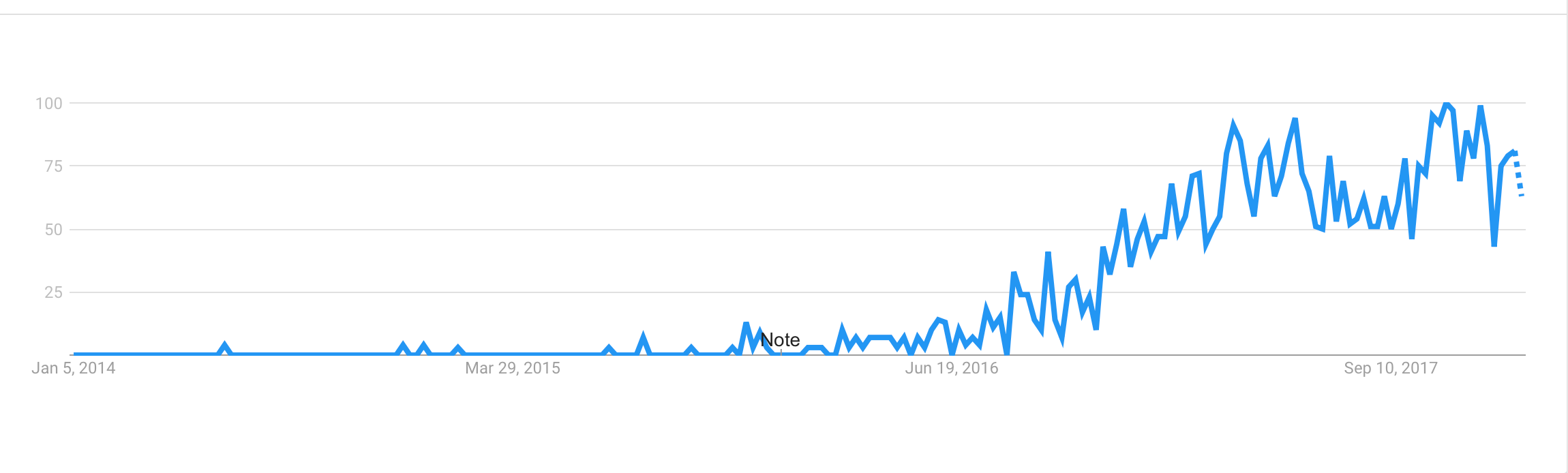Google Trend’s Interest over time for term “Generative Adversarial Networks”

# What’s so magical about GANs?

In short, they belong to the set of algorithms named generative models. These algorithms belong to the field of unsupervised learning, a sub-set of ML which aims to study algorithms that learn the underlying structure of the given data, without specifying a target value. Generative models learn the intrinsic distribution function of the input data p(x) (or p(x,y) if there are multiple targets/classes in the dataset), allowing them to generate both synthetic inputs x’ and outputs/targets y’, typically given some hidden parameters.

In contrast, supervised learning algorithms learn to map a function y’=f(x), given labeled data y. An example of this would be classification, where one could use customer purchase data (x) and the customer respective age (y) to classify new customers. Most of the supervised learning algorithms are inherently discriminative, which means they learn how to model the conditional probability distribution function (p.d.f) p(y|x) instead, which is the probability of a target (age=35) given an input (purchase=milk). Despite the fact that one could make predictions with this probability distribution function, one is not allowed to sample new instances (simulate customers with ages) from the input distribution directly.
Side-note: It is possible to use discriminative algorithms which are not probabilistic, they are called discriminative functions.

GANs they have proven to be really succesfull in modeling and generating high dimensional data, which is why they’ve become so popular. Nevertheless they are not the only types of Generative Models, others include Variational Autoencoders (VAEs) and pixelCNN/pixelRNN and real NVP. Each model has its own tradeoffs.

Some of the most relevant GAN pros and cons for the are:

• They currently generate the sharpest images

# So.. why generative models?

Generative models are one of the most promising approaches to understand the vast amount of data that surrounds us nowadays. According to OpenAI, algorithms which are able to create data might be substantially better at understanding intrinsically the world. The idea that generative models hold a better potential at solving our problems can be illustrated using the quote of one of my favourite physicists.

“What I cannot create, I do not understand.” — Richard P. Feynman

(I strongly suggest reading his book “Surely You’re Joking Mr. Feynman”)

Generative models can be thought as containing more information than their discriminative counterpart/complement, since they also be used for discriminative tasks such as classification or regression (where the target is a continuous value such as). One could calculate the conditional p.d.f p(y|x) needed most of the times for such tasks, by using statistical inference on the joint p.d.f. p(x,y) if it is available in the generative model.

Though generative models work for classification and regression, fully discriminative approaches are usually more successful at discriminative tasks in comparison to generative approaches in some scenarios.

# Use Cases

Among several use cases, generative models may be applied to:

• Generating realistic artwork samples (video/image/audio).

I also found a very long and interesting curated list of awesome GAN applications here.

# 2. Understanding a GAN: Overview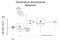Global concept of a GAN

Generative Adversarial Networks are composed of two models:

• The first model is called a Generator and it aims to generate new data similar to the expected one. The Generator could be asimilated to a human art forger, which creates fake works of art.

How do these models interact? Paraphrasing the original paper which proposed this framework, it can be thought of the Generator as having an adversary, the Discriminator. The Generator (forger) needs to learn how to create data in such a way that the Discriminator isn’t able to distinguish it as fake anymore. The competition between these two teams is what improves their knowledge, until the Generator succeeds in creating realistic data.

# Mathematically Modeling a GAN

Though the GANs framework could be applied to any two models that perform the tasks described above, it is easier to understand when using universal approximators such as artificial neural networks.

A neural network G(z, θ₁) is used to model the Generator mentioned above. It’s role is mapping input noise variables z to the desired data space x (say images). Conversely, a second neural network D(x, θ₂) models the discriminator and outputs the probability that the data came from the real dataset, in the range (0,1). In both cases, θᵢ represents the weights or parameters that define each neural network.

As a result, the Discriminator is trained to correctly classify the input data as either real or fake. This means it’s weights are updated as to maximize the probability that any real data input x is classified as belonging to the real dataset, while minimizing the probability that any fake image is classified as belonging to the real dataset. In more technical terms, the loss/error function used maximizes the function D(x), and it also minimizes D(G(z)).

Furthermore, the Generator is trained to fool the Discriminator by generating data as realistic as possible, which means that the Generator’s weight’s are optimized to maximize the probability that any fake image is classified as belonging to the real dataset. Formally this means that the loss/error function used for this network maximizes D(G(z)).

In practice, the logarithm of the probability (e.g. log D(…)) is used in the loss functions instead of the raw probabilies, since using a log loss heavily penalises classifiers that are confident about an incorrect classification.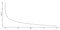Log Loss Visualization: Low probability values are highly penalized

After several steps of training, if the Generator and Discriminator have enough capacity (if the networks can approximate the objective functions), they will reach a point at which both cannot improve anymore. At this point, the generator generates realistic synthetic data, and the discriminator is unable to differentiate between the two types of input.

Since during training both the Discriminator and Generator are trying to optimize opposite loss functions, they can be thought of two agents playing a minimax game with value function V(G,D). In this minimax game, the generator is trying to maximize it’s probability of having it’s outputs recognized as real, while the discriminator is trying to minimize this same value.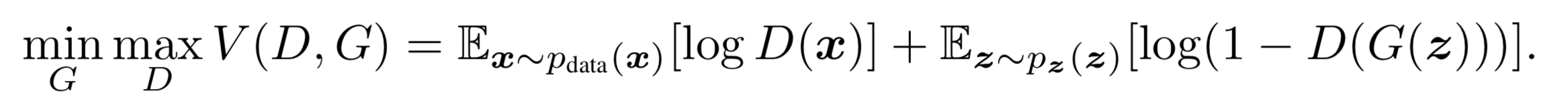Value Function of Minimax Game played by Generator and Discriminator

# Training a GAN

Since both the generator and discriminator are being modeled with neural, networks, agradient-based optimization algorithm can be used to train the GAN. In our coding example we’ll be using stochastic gradient descent, as it has proven to be succesfull in multiple fields.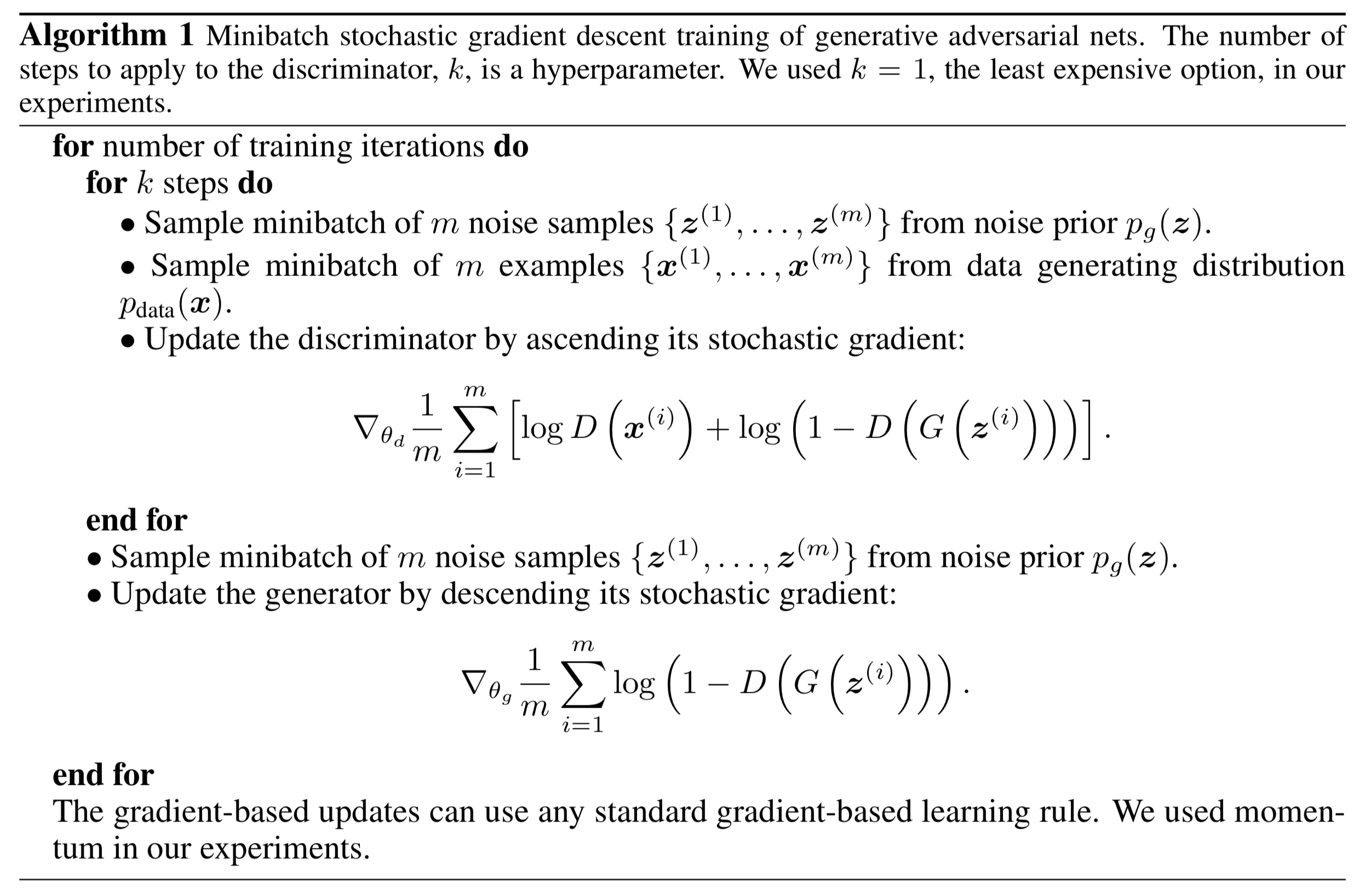Algorithm on how to train a GAN using stochastic gradient descent 

The fundamental steps to train a GAN can be described as following:

1. Sample a noise set and a real-data set, each with size m.

# 3. Coding a GAN

Finally, the moment several of us were waiting for has arrived. 🙌

We’ll implement a GAN in this tutorial, starting by downloading the required libraries.

`pip install torchvision tensorboardx jupyter matplotlib numpy`

In case you haven’t downloaded PyTorch yet, check out their download helper here. Remember that you can also find a TensorFlow example here.

We’ll proceed by creating a file/notebook and importing the following dependencies.

`import torchfrom torch import nn, optimfrom torch.autograd.variable import Variablefrom torchvision import transforms, datasets`

To log our progress, we will import an additional file I’ve created, which will allow us to visualize the training process in console/Jupyter, and at the same time store it in TensorBoard for those who already know how to use it.

`from utils import Logger`

You need to download the file and put it in the same folder where your GAN file will be. It is not necessary that you understand the code in this file, as it is only used for visualization purposes.

The file can be found in any of the following links: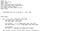Preview of the file we will use for logging.

Dataset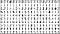MNIST Dataset Samples

The dataset we’ll be using here is LeCunn’s MNIST dataset, consisting of about 60.000 black and white images of handwritten digits, each with size 28x28 pixels². This dataset will be preprocessed according to some useful ‘hacks’ proven to be useful for training GANs.

**Specifically, the input values which range in between [0, 255] will be normalized between -1 and 1. This means the value 0 will be mapped to -1, the value 255 to 1, and similarly all values in between will get a value in the range [-1, 1].

`def mnist_data():    compose = transforms.Compose(        [transforms.ToTensor(),         transforms.Normalize((.5, .5, .5), (.5, .5, .5))        ])    out_dir = './dataset'    return datasets.MNIST(root=out_dir, train=True, transform=compose, download=True)# Load datadata = mnist_data()# Create loader with data, so that we can iterate over itdata_loader = torch.utils.data.DataLoader(data, batch_size=100, shuffle=True)# Num batchesnum_batches = len(data_loader)`

Networks

Next, we’ll define the neural networks, starting with the Discriminator. This network will take a flattened image as its input, and return the probability of it belonging to the real dataset, or the synthetic dataset. The input size for each image will be `28x28=784`. Regarding the structure of this network, it will have three hidden layers, each followed by a Leaky-ReLU nonlinearity and a Dropout layer to prevent overfitting. A Sigmoid/Logistic function is applied to the real-valued output to obtain a value in the open-range (0, 1):

`class DiscriminatorNet(torch.nn.Module):    """    A three hidden-layer discriminative neural network    """    def __init__(self):        super(DiscriminatorNet, self).__init__()        n_features = 784        n_out = 1                self.hidden0 = nn.Sequential(             nn.Linear(n_features, 1024),            nn.LeakyReLU(0.2),            nn.Dropout(0.3)        )        self.hidden1 = nn.Sequential(            nn.Linear(1024, 512),            nn.LeakyReLU(0.2),            nn.Dropout(0.3)        )        self.hidden2 = nn.Sequential(            nn.Linear(512, 256),            nn.LeakyReLU(0.2),            nn.Dropout(0.3)        )        self.out = nn.Sequential(            torch.nn.Linear(256, n_out),            torch.nn.Sigmoid()        )    def forward(self, x):        x = self.hidden0(x)        x = self.hidden1(x)        x = self.hidden2(x)        x = self.out(x)        return xdiscriminator = DiscriminatorNet()`

We also need some additional functionality that allows us to convert a flattened image into its 2-dimensional representation, and another one that does the opposite.

`def images_to_vectors(images):    return images.view(images.size(0), 784)def vectors_to_images(vectors):    return vectors.view(vectors.size(0), 1, 28, 28)`

On the other hand, the Generative Network takes a latent variable vector as input, and returns a 784 valued vector, which corresponds to a flattened 28x28 image. Remember that the purpose of this network is to learn how to create undistinguishable images of hand-written digits, which is why its output is itself a new image.

This network will have three hidden layers, each followed by a Leaky-ReLU nonlinearity. The output layer will have a TanH activation function, which maps the resulting values into the (-1, 1) range, which is the same range in which our preprocessed MNIST images is bounded.

`class GeneratorNet(torch.nn.Module):    """    A three hidden-layer generative neural network    """    def __init__(self):        super(GeneratorNet, self).__init__()        n_features = 100        n_out = 784                self.hidden0 = nn.Sequential(            nn.Linear(n_features, 256),            nn.LeakyReLU(0.2)        )        self.hidden1 = nn.Sequential(                        nn.Linear(256, 512),            nn.LeakyReLU(0.2)        )        self.hidden2 = nn.Sequential(            nn.Linear(512, 1024),            nn.LeakyReLU(0.2)        )                self.out = nn.Sequential(            nn.Linear(1024, n_out),            nn.Tanh()        )    def forward(self, x):        x = self.hidden0(x)        x = self.hidden1(x)        x = self.hidden2(x)        x = self.out(x)        return xgenerator = GeneratorNet()`

We also need some additional functionality that allows us to create the random noise. The random noise will be sampled from a normal distribution with mean 0 and variance 1 as proposed in this link.

`def noise(size):    '''    Generates a 1-d vector of gaussian sampled random values    '''    n = Variable(torch.randn(size, 100))    return n`

Optimization

Here we’ll use `Adam` as the optimization algorithm for both neural networks, with a learning rate of 0.0002. The proposed learning rate was obtained after testing with several values, though it isn’t necessarily the optimal value for this task.

`d_optimizer = optim.Adam(discriminator.parameters(), lr=0.0002)g_optimizer = optim.Adam(generator.parameters(), lr=0.0002)`

The loss function we’ll be using for this task is named Binary Cross Entopy Loss (BCE Loss), and it will be used for this scenario as it resembles the log-loss for both the Generator and Discriminator defined earlier in the post (see Modeling Mathematically a GAN). Specifically we’ll be taking the average of the loss calculated for each minibatch.

`loss = nn.BCELoss()`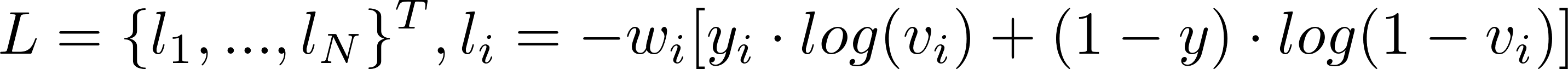Binary Cross Entropy Log. Mean is calculated by computing sum(L) / N.

In this formula the values y are named targets, v are the inputs, and w are the weights. Since we don’t need the weight at all, it’ll be set to wᵢ=1 for all i.

Discriminator Loss: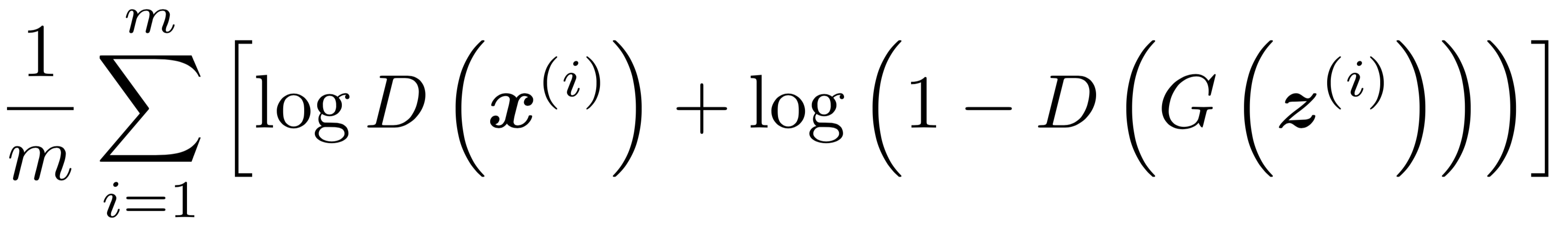Discriminator’s Loss.

If we replace vᵢ = D(xᵢ) and yᵢ=1 ∀ i (for all i) in the BCE-Loss definition, we obtain the loss related to the real-images. Conversely if we set vᵢ = D(G(zᵢ)) and yᵢ=0 ∀ i, we obtain the loss related to the fake-images. In the mathematical model of a GAN I described earlier, the gradient of this had to be ascended, but PyTorch and most other Machine Learning frameworks usually minimize functions instead. Since maximizing a function is equivalent to minimizing it’s negative, and the BCE-Loss term has a minus sign, we don’t need to worry about the sign.

Additionally, we can observe that the real-images targets are always ones, while the fake-images targets are zero, so it would be helpful to define the following functions:

`def ones_target(size):    '''    Tensor containing ones, with shape = size    '''    data = Variable(torch.ones(size, 1))    return datadef zeros_target(size):    '''    Tensor containing zeros, with shape = size    '''    data = Variable(torch.zeros(size, 1))    return data`

By summing up these two discriminator losses we obtain the total mini-batch loss for the Discriminator. In practice, we will calculate the gradients separately, and then update them together.

`def train_discriminator(optimizer, real_data, fake_data):    N = real_data.size(0)    # Reset gradients    optimizer.zero_grad()        # 1.1 Train on Real Data    prediction_real = discriminator(real_data)    # Calculate error and backpropagate    error_real = loss(prediction_real, ones_target(N) )    error_real.backward()    # 1.2 Train on Fake Data    prediction_fake = discriminator(fake_data)    # Calculate error and backpropagate    error_fake = loss(prediction_fake, zeros_target(N))    error_fake.backward()        # 1.3 Update weights with gradients    optimizer.step()        # Return error and predictions for real and fake inputs    return error_real + error_fake, prediction_real, prediction_fake`

Generator Loss: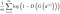Generator’s Loss

Rather than minimizing log(1- D(G(z))), training the Generator to maximize log D(G(z)) will provide much stronger gradients early in training. Both losses may be swapped interchangeably since they result in the same dynamics for the Generator and Discriminator.

Maximizing log D(G(z)) is equivalent to minimizing it’s negative and since the BCE-Loss definition has a minus sign, we don’t need to take care of the sign. Similarly to the Discriminator, if we set vᵢ = D(G(zᵢ)) and yᵢ=1 ∀ i, we obtain the desired loss to be minimized.

`def train_generator(optimizer, fake_data):    N = fake_data.size(0)    # Reset gradients    optimizer.zero_grad()    # Sample noise and generate fake data    prediction = discriminator(fake_data)    # Calculate error and backpropagate    error = loss(prediction, ones_target(N))    error.backward()    # Update weights with gradients    optimizer.step()    # Return error    return error`

Testing

Last thing before we run our algorithm, we want to visualize how the training process develops while our GAN learns. To do so, we will create a static batch of noise, every few steps we will visualize the batch of images the generator outputs when using this noise as input.

`num_test_samples = 16test_noise = noise(num_test_samples)`

Training

Now that we’ve defined the dataset, networks, optimization and learning algorithms we can train our GAN. This part is really simple, since the only thing we’ve got to do is to code in python the pseudocode shown earlier on traning a GAN (see Training a GAN).

We’ll be using all the pieces we’ve coded already, plus the logging file I asked you to download earlier for this procedure:

`# Create logger instancelogger = Logger(model_name='VGAN', data_name='MNIST')# Total number of epochs to trainnum_epochs = 200for epoch in range(num_epochs):    for n_batch, (real_batch,_) in enumerate(data_loader):        N = real_batch.size(0)        # 1. Train Discriminator        real_data = Variable(images_to_vectors(real_batch))        # Generate fake data and detach         # (so gradients are not calculated for generator)        fake_data = generator(noise(N)).detach()        # Train D        d_error, d_pred_real, d_pred_fake = \              train_discriminator(d_optimizer, real_data, fake_data)        # 2. Train Generator        # Generate fake data        fake_data = generator(noise(N))        # Train G        g_error = train_generator(g_optimizer, fake_data)        # Log batch error        logger.log(d_error, g_error, epoch, n_batch, num_batches)        # Display Progress every few batches        if (n_batch) % 100 == 0:             test_images = vectors_to_images(generator(test_noise))            test_images = test_images.data            logger.log_images(                test_images, num_test_samples,                 epoch, n_batch, num_batches            );            # Display status Logs            logger.display_status(                epoch, num_epochs, n_batch, num_batches,                d_error, g_error, d_pred_real, d_pred_fake            )`

And that’s it, we’ve made it! 🎊

# Results

In the beginning the images generated are pure noise:

But then they improve,

Until you get pretty good syntethic images,

It is also possible to visualize the learning process. As you can see in the next figures, the discriminator error is very high in the beginning, as it doesn’t know how to classify correctly images as being either real or fake. As the discriminator becomes better and its error decreases to about .5 at step 5k, the generator error increases, proving that the discriminator outperforms the generator and it can correctly classify the fake samples. As time passes and training continues, the generator error lowers, implying that the images it generates are better and better. While the generator improves, the discriminator’s error increases, because the synthetic images are becoming more realistic each time.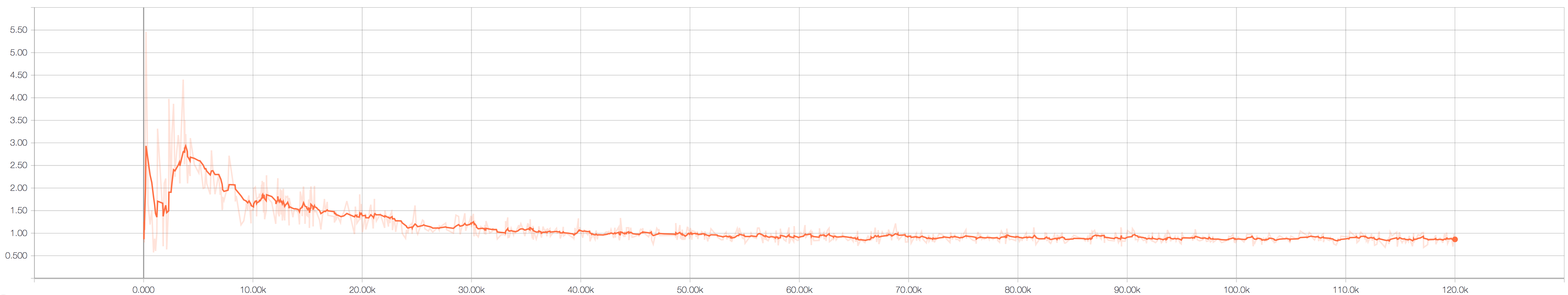Generator’s Error through Time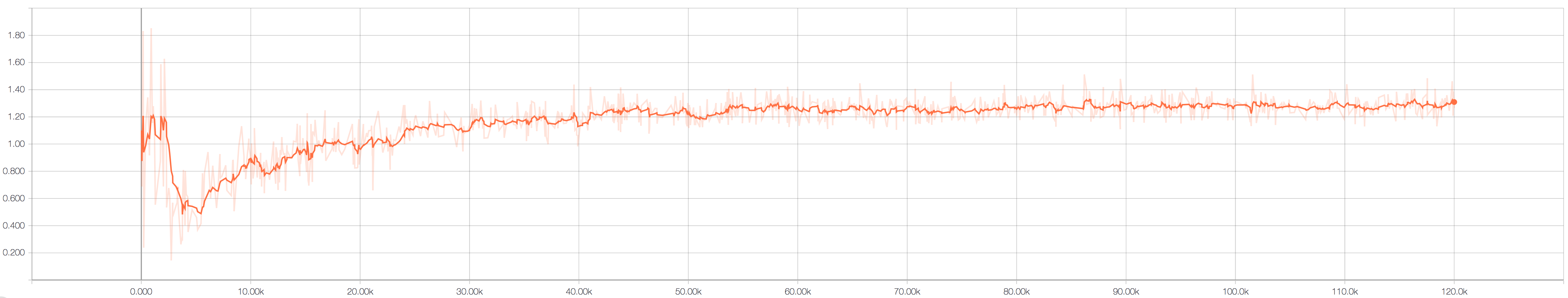Discriminator’s Error through Time

You can also check out the notebook named Vanilla Gan PyTorch in this link and run it online. You may also download the output data.

• runs/ folder contains the tensor board data.

# Conclusions

In this blog post I have introduced Generative Adversarial Networks. We started by learning what kind of algorithms they are, and why they’re so relevant nowadays. Next we explored the parts that conform a GAN and how they work together. Finally we finished linking the theory with the practice by programming with a fully working implementation of a GAN that learned to create synthetic examples of the MNIST dataset.

Now that you’ve learned all of this, next steps would be to keep on reading and learning about the more advanced GAN methods that I listed in the Further Reading Section. As mentioned earlier, I’ll keep writing these kind of tutorials to make it easier for enthusiasts to learn Machine Learning in a practical way, and learning required maths in the way.

# Further Reading/Watching

Thanks for reading this post until the end, I’m really glad to find people who’re as motivated as I am about science (specifically CS and AI).

Make sure to like/share this post 😊 , and comment your experience reading it!

Feel free to leave any questions and suscribe for future state-of-the-art ML explanations.

GitHub: diegoalejogm
LinkedIn: diegoalejogm

# References

 Jun-Yan Zhu, Taesung Park, Phillip Isola, Alexei A. Efros, Unpaired Image-to-Image Translation using Cycle-Consistent Adversarial Networks, 2018, https://arxiv.org/abs/1703.10593

 Ian J. Goodfellow, Jean Pouget-Abadie, Mehdi Mirza, Bing Xu, David Warde-Farley, Sherjil Ozair, Aaron Courville, Yoshua Bengio, Generative Adversarial Networks, 2014, https://arxiv.org/abs/1406.2661

## AI Society

Artificial Intelligence Hacking Group

## AI Society

Artificial Intelligence Hacking Group

Written by

## Diego Gomez Mosquera

ML Engineer 👾 Googler 🎈 Passionate about science 🔭 Find me at: diegogom.com## AI Society

Artificial Intelligence Hacking Group

## More From Medium

Medium is an open platform where 170 million readers come to find insightful and dynamic thinking. Here, expert and undiscovered voices alike dive into the heart of any topic and bring new ideas to the surface. Learn more

Follow the writers, publications, and topics that matter to you, and you’ll see them on your homepage and in your inbox. Explore

If you have a story to tell, knowledge to share, or a perspective to offer — welcome home. It’s easy and free to post your thinking on any topic. Write on Medium